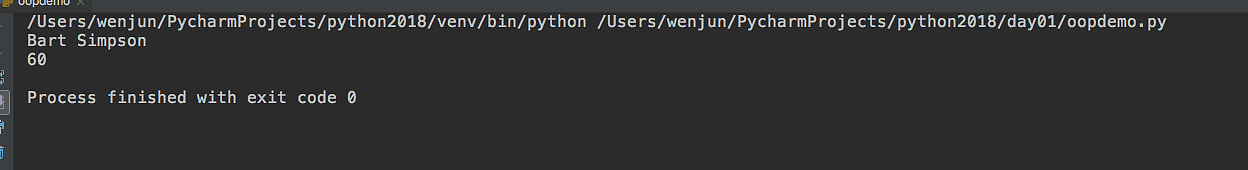# python 面向对象``````std1 = { 'name': 'Michael', 'score': 98 }
std2 = { 'name': 'Bob', 'score': 81 }``````

``````def print_score(std):
print('%s: %s' % (std['name'], std['score']))``````

``````class Student(object):

def __init__(self,name,score):
self.name = name
self.score = score

def print_score(self):
print('%s: %s' %(self.name,self.score))``````

``````bart = Student('Bart Simpson',59)
bart.print_score()``````

# 类和实例

``````class Student(object):
pass``````

class后面紧接着是类名，即Student，类名通常是大写开头的单词，紧接着是(object)，表示该类是从哪个类继承下来的，通常，如果没有合适的继承类，就使用object类，这是所有类最终都会继承的类。

``````class Student(object):

def __init__(self, name, score):
self.name = name
self.score = score``````

init方法的第一个参数永远是self，表示创建的实例本身，因此，在init方法内部，就可以把各种属性绑定到self，因为self就指向创建的实例本身。

# 数据封装

``````class Student(object):

def __init__(self,name,score):
self.name = name
self.score = score

def print_score(self):
print('%s: %s' %(self.name,self.score))

if self.score >= 90:
return 'A'
elif self.score >=60:
return 'B'
else:
return 'C'

bart = Student('Bart Simpson',59)

# 访问限制

``````def __init__(self,name,score):
self.__name = name
self.__score = score

def print_score(self):
print('%s: %s' %(self.name,self.score))``````

``````class Student(object):

def __init__(self,name,score):
self.__name = name
self.__score = score

def get_name(self):
return self.__name

def get_score(self):
return self.__score
def print_score(self):
print('%s: %s' %(self.name,self.score))

if self.score >= 90:
return 'A'
elif self.score >=60:
return 'B'
else:
return 'C'

bart = Student('Bart Simpson',59)
print(bart.get_name())``````

``````class Student(object):

def __init__(self,name,score):
self.__name = name
self.__score = score

def get_name(self):
return self.__name

def get_score(self):
return self.__score
def print_score(self):
print('%s: %s' %(self.name,self.score))

def set_score(self,score):
self.__score = score

if self.score >= 90:
return 'A'
elif self.score >=60:
return 'B'
else:
return 'C'

bart = Student('Bart Simpson',59)
print(bart.get_name())
bart.set_score(60)
print(bart.get_score())    ````````````class Student(object):
……

def set_score(self,score):
if 0<=score<=100:
self.__score = score
else:

bart = Student('Bart Simpson',59)
print(bart.get_name())
bart.set_score(101)
print(bart.get_score())````````````>>> bart._Student__name
'Bart Simpson'``````